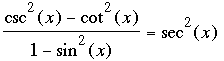SEARCH HOMEMath Central Quandaries & QueriesQuestion from Christine, a student: I need help with this whole paper here is one problem csc^2x-cot^2x/1-sin^2x=sec^2x by the ^2 i mean squared pleeaseHi Christine,

I would takeand write each of the trig functions in terms of sin(x) and cos(x). For example csc(x) = 1/sin(x). Simplify the left side using the fact that sin2(x) + cos2(x) = 1.

PennyMath Central is supported by the University of Regina and The Pacific Institute for the Mathematical Sciences.